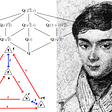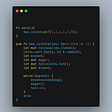# Intuition behind correlation

1. It cancels the effect of units of each column in the bivariate data series and hence makes correlation independent of units so that we can compare any series
2. It brings the correlation to the range of -1 to 1 which provides a universal scale for comparison of bivariate data series
3. Since standard deviation is always positive, it does not change the sign of any term in the formula of covariance

# Properties of correlation

1. It is symmetric which means that correlation between x and y is same as the correlation between y and x
2. It is always between -1 and 1
3. Correlation coefficient is independent of scale change and origin change which means that correlation does not change when you multiply or divide each element of the series with a particular number or you add or subtract each element of the series by a particular number

# Inference of correlation

1. If the correlation coefficient is close to -1 it means that the value of x decreases with increase in value of y. This implies strong negative correlation
2. If the correlation coefficient is close to 0 it means that the value of x is independent of value of y. This implies no correlation
3. If the correlation coefficient is close to 1 it means that the value of x increases with increase in value of y. This implies strong positive correlation

# Demerits of correlation

--

--

--

## More from Aayushmaan Jain

A data science enthusiast currently doing bachelor's degree in data science

Love podcasts or audiobooks? Learn on the go with our new app.

## Galois theory for non-mathematicians## HOW TO FIND YOUR ANGEL NUMBER- 3 WAYS TO KNOW FOR SURE## Maximum Number of Coins You Can Get## How to Calculate the Fibonacci Sequence in Logarithmic Time## What is Googol The Number? How Is Related to Google?## Like Love, Mathematicians Win in the End, and Math Quotes## Infinity and I## Variational Quantum Eigensolver from Scratch: Finding ground-state energy of Hamiltonian## Aayushmaan Jain

A data science enthusiast currently doing bachelor's degree in data science

## Data Transformation in Machine Learning Part-II## DIY Gradient Descending Linear Regression## One easy way to split data into train and test sets for machine learning## Predicting Personal Loan defaulter